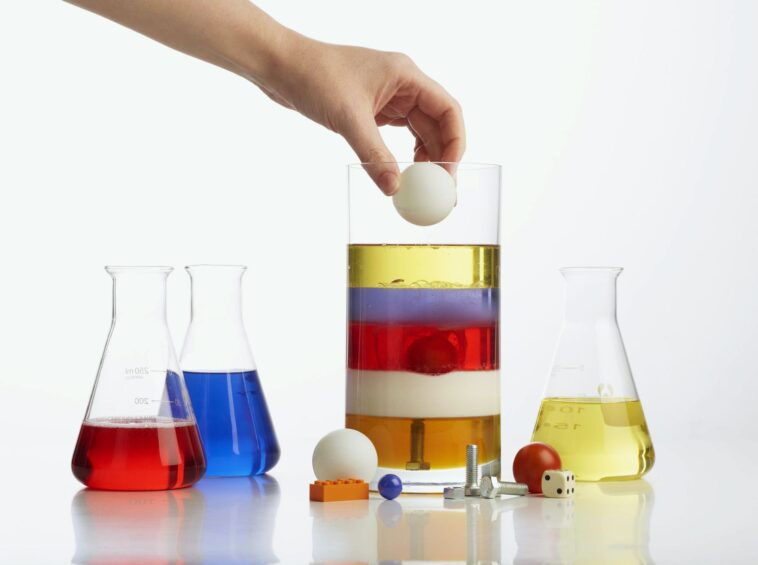# What We Can Learn by Measuring Density?Density is an important concept in various sciences, especially physics and chemistry. It is a basic property of matter that has many applications. By understanding it, we can learn about the composition of various substances, the behavior of gasses, and even the Earth’s climate. In this blog post, we will explore what density is and how it can be measured. We will also discuss some of the important applications of density measurements.

## How to Measure Density?

Density is a measure of how much mass an object has in a given volume. In other words, it is a measure of how “tightly packed” an object is. There are many factors to consider when measuring it, and the most important thing is to use the right tools for the job.

The first step is to determine the volume of the object in question. This can be done by using a simple ruler or measuring cup. Once you have the object’s volume, you need to find its mass. The easiest way to do this is to weigh the object on a scale.

Now that you have both the mass and volume of the object, you can calculate its density. The density of an object is equal to its mass divided by its volume. For example, if an object has a mass of 10 grams and a volume of 2 cubic centimeters, its density would be 5 grams per cubic centimeter. Using a density and pressure calculator you can easily calculate it.

It is an important property of matter that tells us how much space an object takes up for a given mass. It is a key concept in fields such as physics, engineering, and even medicine. By understanding how to measure density, we can learn a lot about the world around us.

## The Importance of Measuring Density

This is one of the fundamental properties of matter. It tells us how much mass is contained in a given volume. The density of an object can tell us a lot about what it’s made of. For example, rocks are usually denser than other materials like air or water. This is because rocks are made up of dense materials like minerals and metals. So, when you measure the density of rock, you’re really measuring the amount of these dense materials it contains.

## What Can We Learn from Measuring Density?

There are many things that we can learn from measuring density.

For one, we can learn the composition of a given substance. This is because different substances have different densities. For example, water has a density of 1 g/mL, while oil has a density of 0.9 g/mL. This means that if we measure the density of a substance, we can determine what it is made of.

Additionally, measuring it can tell us how much mass is present in a given volume. This is because density is defined as mass per unit volume. So, if we know the density of an object, we can calculate its mass by multiplying its density by its volume. This information can be useful in many applications, such as determining the weight of an object or calculating the amount of material needed for a specific project.

## Conclusion

Density is an important property of matter that helps us understand the physical world around us. By measuring it, we can learn about the composition of objects and how they interact with their surroundings. It also allows us to calculate the mass of an object, which is essential in many fields such as engineering and physics. In conclusion, density is a fundamental property of matter that provides valuable information about the physical world.### Cost of Living Crisis: 5 Ways to Cope